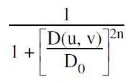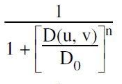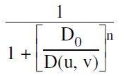## Image-Processing

 Question 1
Hidden surface removal problem with minimal 3D pipeline can be solved with
 A Painter's algorithm B Window Clipping algorithm C Brute force rasterization algorithm D Flood fill algorithm
Image-Processing       Window-Clipping-Algorithm       NTA-UGC-NET 2021 Dec & 2022 June Paper-2
Question 1 Explanation:
Hidden surface removal problem with minimal 3D pipeline can be solved with Window Clipping algorithm.
The z-buffer algorithm is the most widely used method for solving the hidden surface problem. It has the following major advantages over other hidden surface removal algorithms:
-No sorting is required. Models can be rendered in any order.
-No geometric intersection calculations are required. The algorithm produces the correct output even for intersecting or overlapping triangles.
-The algorithm is very simple to implement.

Disadvantages of the z-buffer algorithm include:
-A z-buffer requires a non-trivial amount of memory. For example, assuming each value in a z-buffer is a 32 bit floating point value, a rendered image that is 1024x768 pixels requires 3MB of memory to store its z-buffer.
-Every pixel of every primitive element must be rendered, even if many of them never write their color to the frame buffer.
-If two primitives are in exactly the same place in 3D space, as their positions are interpolated across their respective surfaces, the z values for each object will typically be different by a very small amount due to floating-point round-off errors. These small differences will alternate between primitives for adjacent pixels resulting in random and weird patterns in a rendering. This is called “z-fighting” and it can be avoided by never placing two primitives in the same location in 3D space.
 Question 2
What does a pixel mask mean?
 A string containing only 1’s B string containing only 0’s C string containing two 0’s D string containing 1’s and 0’s
Image-Processing       Pixel-Mask       ISRO CS 2014       Video-Explanation
Question 2 Explanation:
Pixel mask: when a given image is intended to be placed over a background, the transparent areas can be specified through a binary mask.
→ This way, for each intended image there are actually two bitmaps:
1. Actual image, in which the unused areas are given a pixel value with all bits set to 0s.
2. Additional mask, in which the correspondent image areas are given a pixel value of all bits set to 0s and the surrounding areas a value of all bits set to 1s.
In the sample at right, black pixels have the all-zero bits and white pixels have the all-one bits.
 Question 3
If the frame buffer has 8 bits per pixel and 8 bits are allocated for each of the R, G, B components, what would be the size of the lookup table?
 A 24 bytes B 1024 bytes C 768 bytes D 256 bytes
Image-Processing       Frame-buffer       ISRO CS 2013       Video-Explanation
Question 3 Explanation:
Given data,
Frame buffer =8 bits per pixel
Each component(R,G,B)= 8 bitsQuestion 4
Consider an uncompressed stereo audio signal of CD quality which is sampled at 44.1 kHz and quantized using 16 bits. What is required storage space if a compression ratio of 0.5 is achieved for 10 seconds of this audio?
 A 172 KB B 430 KB C 860 KB D 1720 KB
Image-Processing       Multimedia       ISRO CS 2015       Video-Explanation
Question 4 Explanation:
Given frequency = 44.1 kHz = 44100 bits per second and compression ratio = 0.5
This is stereo audio signal, it will need 2 channels. 16 bits used for quantized (converting to MP3)

Bit Rate = number channels * bits* frequency =2 x16 x 44100 = 1411200 bps

Compressed Bit Rate = 0.5x 1411200= 705600 bps ( It is for one second)
For 10 seconds,
Bit rate = 10 x 705600 bits
= 7056000bits
=882000 Bytes (7056000/8 & 1 Byte =8 bits)
= 882 KB (882000/1000& 1 KB=1000 bytes)
 Question 5The Laplacian of an image at all points (x, y) can be implemented by convolving the image with spatial mask. Which of the following can be used as the spatial mask ?
 A only S1 B only S2 C Both S1 and S2 D None of these
Image-Processing       convolution       UGC NET CS 2014 Dec - paper-3
 Question 6
Given a simple image of size 10 × 10 whose histogram models the symbol probabilities and is given byThe first order estimate of image entropy is maximum when
 A a = 0, b = 0, c = 0, d = 1 B a =1/2, b =1/2, c = 0, d = 0 C a =1/3, b =1/3, c =1/3, d = 0 D a =1/4, b =1/4, c =1/4, d =1/4
Image-Processing       Entropy       UGC NET CS 2014 Dec - paper-3
 Question 7
A Butterworth lowpass filter of order n, with a cutoff frequency at distance D0 from the origin, has the transfer function H(u, v) given by
 ABCDImage-Processing       Filters       UGC NET CS 2014 Dec - paper-3
 Question 8
Blind image deconvolution is ____________.
 A Combination of blur identification and image restoration B Combination of segmentation and classification C Combination of blur and non-blur image D None of the above
Image-Processing       deconvolution       UGC NET CS 2015 Dec - paper-3
Question 8 Explanation:
Blind deconvolution: a deconvolution technique that permits recovery of the target scene from a single or set of "blurred" images in the presence of a poorly determined or unknown point spread function (PSF). Any blurred image can be given as input to blind deconvolution algorithm, it can deblur the image.
 Question 9
Which of the following is used for the boundary representation of an image object?
 A Quad Tree B Projections C Run length coding D Chain codes
Image-Processing       Chain-Codes       UGC NET CS 2016 July- paper-3
Question 9 Explanation:
→ Chain codes is used for the boundary representation of an image object.

→ A chain code is a lossless compression algorithm for monochrome images. The basic principle of chain codes is to separately encode each connected component, or "blob", in the image.

→ For each such region, a point on the boundary is selected and its coordinates are transmitted. The encoder then moves along the boundary of the region and, at each step, transmits a symbol representing the direction of this movement.

 Question 10
Which of the following is not used in standard JPEG image compression?
 A Huffman coding B Run length encoding C Zig-zag scan D K-L Transform
Image-Processing       Image-Compression       UGC NET CS 2016 Aug- paper-3
Question 10 Explanation:
→ Huffman coding, Run length encoding and ZIg-zag scan is used to compress the image.
K-L Transform is a representation of a stochastic process as an infinite linear combination of orthogonal functions, analogous to a Fourier series representation of a function on a bounded interval. The transformation is also known as Hotelling transform and eigenvector transform, and is closely related to principal component analysis (PCA) technique widely used in image processing and in data analysis in many fields.
 Question 11
If the histogram of an image is clustered towards origin on X-axis of a histogram plot then it indicates that the image is ______.
 A Dark B Good contrast C Bright D Very low contrast
Image-Processing       Histogram       UGC NET CS 2016 Aug- paper-3
Question 11 Explanation:
→ If the histogram of an image is clustered towards origin on X-axis of a histogram plot then it indicates that the image is dark.
→ An image histogram is a type of histogram that acts as a graphical representation of the tonal distribution in a digital image. It plots the number of pixels for each tonal value. By looking at the histogram for a specific image a viewer will be able to judge the entire tonal distribution at a glance.
→ The histogram for a very dark image will have the majority of its data points on the left side and center of the graph. Conversely, the histogram for a very bright image with few dark areas and/or shadows will have most of its data points on the right side and center of the graph.
 Question 12
From the given data below :
a b b a a b b a a b
which one of the following is not a word in the dictionary created by LZ-coding (the initial words are a, b)?
 A a b B b b C b a D b a a b E None of the above
Image-Processing       LZ-Coding       UGC NET CS 2017 Jan- paper-3
Question 12 Explanation:
Lempel–Ziv–Welch is a universal lossless data compression algorithm created by Abraham Lempel, Jacob Ziv, and Terry Welch
abbaabbaab
⇒ Dictionary created by L2 - coding will contain words:
a | b | ba | ab | baa | b
b - already present in dictionary
So the words are a, b, ba, ab, baa.
⇒ Hence ‘B’ and ‘D’ both are not present in the dictionary.
Note: (B) and (D) is correct answers
There are 12 questions to complete.

Register Now